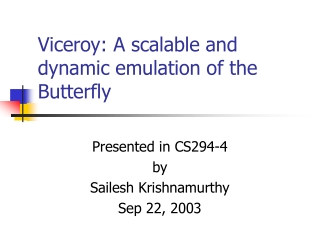Download PresentationViceroy: A scalable and dynamic emulation of the Butterfly

# Viceroy: A scalable and dynamic emulation of the Butterfly

Télécharger la présentation## Viceroy: A scalable and dynamic emulation of the Butterfly

- - - - - - - - - - - - - - - - - - - - - - - - - - - E N D - - - - - - - - - - - - - - - - - - - - - - - - - - -
##### Presentation Transcript

1. Viceroy: A scalable and dynamic emulation of the Butterfly Presented in CS294-4 by Sailesh Krishnamurthy Sep 22, 2003

2. Viceroy • Goals: An overlay routing network with • Logarithmic path lengths • Constant join/leave cost • Balanced congestion (~ log(n)/n) • Keys, servers mapped to unit ring [0,1) • In Chord: each node has all log(n) links • In Viceroy: • Each node has one log(n) link • A link to 1/2k distance points to a node with a link to 1/2(k+1)distance

3. Viceroy Topology • Each node has a level between 1..log(n) • A `level-k’ node has • “right child”: A long-range link to distance 1/2k (approx.), to a level-(k+1)’ node • “left child”: A local link to `level-(k+1)’ node • Level ring links (pred,succ of the same level) • “up”: A local link to ‘level-(k-1)’ node • Ring links (pred,succ on the ring)

4. y 0 x 1 Level 1 Level 2 Level 3 Viceroy Topology

5. Distributed level selection • Select Level (s) • Let n0 = 1/d(s,succ(s)) • Select a level among [1 … log(n0)] uniformly at random • Sanity • When n servers present, then w.h.p. every server estimates: log(n/2logn)<=log(n0)<=3logn • Any level l <log(n/2logn) is “sane”

6. Lookup target in Viceroy • Three phases: • Proceed to root: up-links to a `level-1’ node • for level k = 1..log(n) • If distance < 1/2k use down-left (short link) • If distance >= 1/2k use down-right (long link) • If reqd down link doesn’t exist (or if you overshoot target) break to next phase • Traverse the ring (pred/succ links, whichever is closer)

7. Analysis • Constant out-degree • Expected constant in-degree • log(n ) w.h.p. • O(log(n )) lookup steps w.h.p • log(n ) to `level-1’ node • log(n ) for binary search • log(n ) for final local search • Congestion: • Expected log(n )/n lookup load • O(log2(n )/n) w.h.p.

8. Improving Lookup • Simple lookup - third phase may be too long • May be log2n links to traverse :-( • Fancy lookup • Use a combination of global and level rings to get a dilation of log(n) w.h.p. • Greedy approach - use the level links if you are still too far away from the target.

9. What happens on node leave? • Problem: we have constant out-degree, but even with a sparse network we could have log(n) in-degree on average • Solution: “buckets” - extra background process • Idea: improve identity/level-selection so that we have constant number of nodes in each stretch of (log(n)/n) nodes • Maintain ~n buckets of ~log(n) contiguous non-overlapping nodes.

10. Bucket properties • Size: always θ(log(n)) • Merge with neighbouring bucket when size falls below log(n) • Split bucket when size grows above clog(n) • Diversity in bucket • Each level in [1..log(n)] is represented by (1,c) nodes. Claim: this limits the indegree to 2c - how ?

11. Some comparisons SkipNets log(n) log(n) ?? SkipList

12. Some questions • How important are const degree networks ? • Dilation and congestion same as chord • Depends on bucket mechanism • What about fault tolerance ?